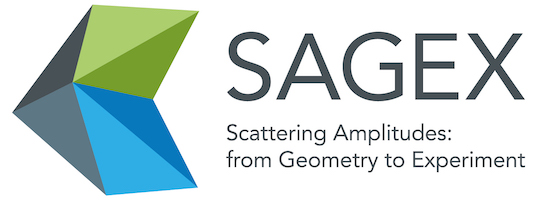# Massive 2- and 3-loop corrections to hard scattering processes in QCD

## Abstract

This thesis deals with calculations of higher-order corrections in perturbative quantum chromodynamics (QCD). The two-mass contributions to the 3-loop, polarized twist-two operator matrix elements (OMEs) Qq and ggQ are calculated. The N-space result for ggQ is obtained analytically as a function of the quark mass ratio, which for Qq is not yet possible. In the z-space representation, one obtains for both matrix elements semi-analytical representations in terms of iterated integrals, whereby for reasons of efficiency an additional integral is necessary for some terms.

These universal (process-independent) massive OMEs govern the asymptotic behaviour of the Wilson coefficients in deep-inelastic scattering at large virtualities Q2 ≫ m2c,b, with mc,b the charm and bottom quark masses. These corrections are also required to define the variable flavour number scheme. This scheme describes the transition from massive quark corrections to the massless ones for very high momentum scales, which is relevant to the description of collider data.

In the single-mass, polarized case, we derive the logarithmic corrections for the Wilson coef- ficients of the structure function g1 in the asymptotic region Q2 ≫ m2c,b. This is done using the known OMEs and massless Wilson coefficients, using the renormalization group equations.

For the non-singlet structure functions $F_2^{NS}$ and $g_1^{NS}$ we revisit the scheme-invariant evolution operator known for massless quarks and extend it to the massive case with single- and two-mass corrections. In this case, the evolution can effectively be described up to O($a^3_s$) in the Wilson coefficients, where as = αs/(4π) denotes the strong coupling constant. The influence of the hitherto not fully known 4-loop non-singlet anomalous dimension can be described effectively. It turns out that the effect of the theory error in question can be completely controlled. A representation by a Pad ́e approximant proves to be sufficient.

We consider the class of functions of multivariate hypergeometric series and study systems of differential equations obeyed by them. We describe an algorithmic method to solve some classes of such differential systems which delivers a hypergeometric series solution having nested hypergeometric products as summand; we discuss the relationship between these products and Pochhammer symbols. For a number of classical hypergeometric series we derive differential systems and their associated difference equations. We present some examples of series expan- sions of such functions and of the mathematical objects which arise therein. We also present a Mathematica package which implements algorithms related to the solution of partial linear dif- ference equations, focusing in particular on bounding the degree of the denominator of solutions which are rational functions. These methods are of particular importance when solving multi-leg calculations for Feynman diagrams, but also come into play when hypergeometric methods for multi-loop integrals are used.

We describe a numerical implementation of an N-space library for the calculation of scal- ing violations for structure functions, which can perform the evolution of parton distribution functions up to NNLO from a parametrization chosen by the user, and encodes massless and massive Wilson coefficients for the structure functions $F_2$ and $g_1$ in the case of photon exchange, and for the structure functions in the case of charged-current exchange. The library contains analytic continuation of the relevant harmonic sums in Mellin-space up to weight 5 and many weight-6 harmonic sums. The numerical representation in x space is performed by contour integration around the singularities of the solution of the evolution equations in N space.

Funded in part by the European Union’s Horizon 2020 Framework Program under the Marie Skłodowska-Curie grant agreement No. 764850 (SAGEX).Tags:

Categories:

Updated: